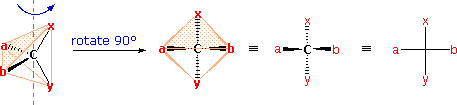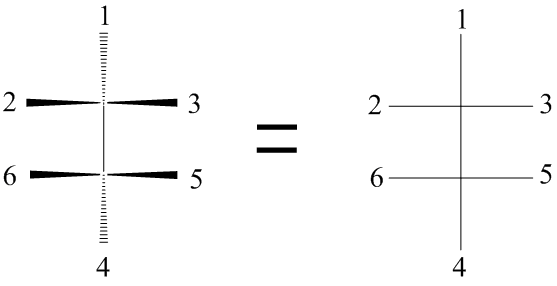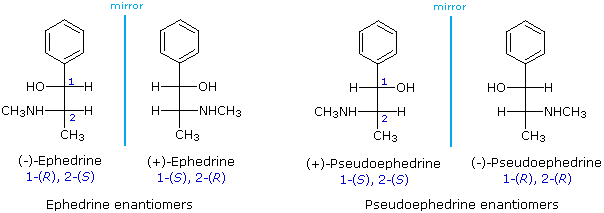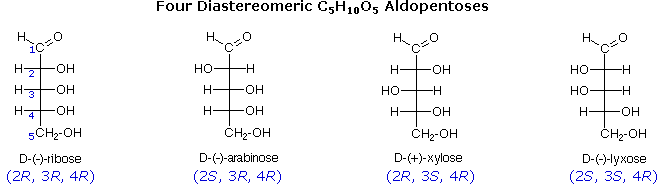# 24.2: Fischer Projections

Objectives

After completing this section, you should be able to

1. draw the Fischer projection of a monosaccharide, given its wedge‑and‑broken‑line structure or a molecular model.
2. draw the wedge‑and‑broken‑line structure of a monosaccharide, given its Fischer projection or a molecular model.
3. construct a molecular model of a monosaccharide, given its Fischer projection or wedge‑and‑broken‑line structure.

Key Terms

Make certain that you can define, and use in context, the key term below.

• Fischer projection

Study Notes

When studying this section, use your molecular model set to assist you in visualizing the structures of the compounds that are discussed. It is important that you be able to determine whether two apparently different Fischer projections represent two different structures or one single structure. Often the simplest way to check is to construct a molecular model corresponding to each projection formula, and then compare the two models.

The problem of drawing three-dimensional configurations on a two-dimensional surface, such as a piece of paper, has been a long-standing concern of chemists. The wedge and hatched line notations we have been using are effective, but can be troublesome when applied to compounds having many chiral centers. As part of his Nobel Prize-winning research on carbohydrates, the great German chemist Emil Fischer, devised a simple notation that is still widely used. In a Fischer projection drawing, the four bonds to a chiral carbon make a cross with the carbon atom at the intersection of the horizontal and vertical lines. The two horizontal bonds are directed toward the viewer (forward of the stereogenic carbon). The two vertical bonds are directed behind the central carbon (away from the viewer). Since this is not the usual way in which we have viewed such structures, the following diagram shows how a stereogenic carbon positioned in the common two-bonds-in-a-plane orientation ( x–C–y define the reference plane ) is rotated into the Fischer projection orientation (the far right formula). When writing Fischer projection formulas it is important to remember these conventions. Since the vertical bonds extend away from the viewer and the horizontal bonds toward the viewer, a Fischer structure may only be turned by 180º within the plane, thus maintaining this relationship. The structure must not be flipped over or rotated by 90º.In the above diagram, if x = CO2H, y = CH3, a = H & b = OH, the resulting formula describes (R)-(–)-lactic acid. The mirror-image formula, where x = CO2H, y = CH3, a = OH & b = H, would, of course, represent (S)-(+)-lactic acid.

The Fischer Projection consists of both horizontal and vertical lines, where the horizontal lines represent the atoms that are pointed toward the viewer while the vertical line represents atoms that are pointed away from the viewer. The point of intersection between the horizontal and vertical lines represents the central carbon.Using the Fischer projection notation, the stereoisomers of 2-methylamino-1-phenylpropanol are drawn in the following manner. Note that it is customary to set the longest carbon chain as the vertical bond assembly.The usefulness of this notation to Fischer, in his carbohydrate studies, is evident in the following diagram. There are eight stereoisomers of 2,3,4,5-tetrahydroxypentanal, a group of compounds referred to as the aldopentoses. Since there are three chiral centers in this constitution, we should expect a maximum of 23 stereoisomers. These eight stereoisomers consist of four sets of enantiomers. If the configuration at C-4 is kept constant (R in the examples shown here), the four stereoisomers that result will be diastereomers. Fischer formulas for these isomers, which Fischer designated as the "D"-family, are shown in the diagram. Each of these compounds has an enantiomer, which is a member of the "L"-family so, as expected, there are eight stereoisomers in all. Determining whether a chiral carbon is R or S may seem difficult when using Fischer projections, but it is actually quite simple. If the lowest priority group (often a hydrogen) is on a vertical bond, the configuration is given directly from the relative positions of the three higher-ranked substituents. If the lowest priority group is on a horizontal bond, the positions of the remaining groups give the wrong answer (you are in looking at the configuration from the wrong side), so you simply reverse it.The aldopentose structures drawn above are all diastereomers. A more selective term, epimer, is used to designate diastereomers that differ in configuration at only one chiral center. Thus, ribose and arabinose are epimers at C-2, and arabinose and lyxose are epimers at C-3. However, arabinose and xylose are not epimers, since their configurations differ at both C-2 and C-3.

## How to make Fischer Projections

To make a Fischer Projection, it is easier to show through examples than through words. Lets start with the first example, turning a 3D structure of ethane into a 2D Fischer Projection.

Example 25.2.1

Start by mentally converting a 3D structure into a Dashed-Wedged Line Structure. Remember, the atoms that are pointed toward the viewer would be designated with a wedged lines and the ones pointed away from the viewer are designated with dashed lines.

Notice the red balls (atoms) in Figure A above are pointed away from the screen. These atoms will be designated with dashed lines like those in Figure B by number 2 and 6. The green balls (atoms) are pointed toward the screen. These atoms will be designated with wedged lines like those in Figure B by number 3 and 5. The blue atoms are in the plane of the screen so they are designated with straight lines.

Now that we have our Dashed- Wedged Line Structure, we can convert it to a Fischer Projection. However, before we can convert this Dashed-Wedged Line Structure into a Fischer Projection, we must first convert it to a “flat” Dashed-Wedged Line Structure. Then from there we can draw our Fischer Projection. Lets start with a more simpler example. Instead of using the ethane shown in Figure A and B, we will start with a methane. The reason being is that it allows us to only focus on one central carbon, which make things a little bit easier.